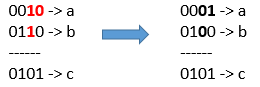Formatted question description: https://leetcode.ca/all/1318.html

# 1318. Minimum Flips to Make a OR b Equal to c (Medium)

Given 3 positives numbers a, b and c. Return the minimum flips required in some bits of a and b to make ( a OR b == c ). (bitwise OR operation).
Flip operation consists of change any single bit 1 to 0 or change the bit 0 to 1 in their binary representation.

Example 1:Input: a = 2, b = 6, c = 5
Output: 3
Explanation: After flips a = 1 , b = 4 , c = 5 such that (a OR b == c)

Example 2:

Input: a = 4, b = 2, c = 7
Output: 1


Example 3:

Input: a = 1, b = 2, c = 3
Output: 0


Constraints:

• 1 <= a <= 10^9
• 1 <= b <= 10^9
• 1 <= c <= 10^9

Related Topics:
Bit Manipulation

## Solution 1.

// OJ: https://leetcode.com/problems/minimum-flips-to-make-a-or-b-equal-to-c/

// Time: O(1)
// Space: O(1)
class Solution {
public:
int minFlips(int a, int b, int c) {
int ans = 0;
for (int i = 0; i < 31; ++i) {
if ((c >> i) & 1) ans += ((a >> i) & 1) == 0 && ((b >> i) & 1) == 0;
else ans += ((a >> i) & 1) + ((b >> i) & 1);
}
return ans;
}
};


Java

class Solution {
public int minFlips(int a, int b, int c) {
int flips = 0;
StringBuffer aBinarySB = new StringBuffer(Integer.toBinaryString(a));
StringBuffer bBinarySB = new StringBuffer(Integer.toBinaryString(b));
StringBuffer cBinarySB = new StringBuffer(Integer.toBinaryString(c));
while (aBinarySB.length() < 32)
aBinarySB.insert(0, '0');
while (bBinarySB.length() < 32)
bBinarySB.insert(0, '0');
while (cBinarySB.length() < 32)
cBinarySB.insert(0, '0');
for (int i = 0; i < 32; i++) {
char aChar = aBinarySB.charAt(i);
char bChar = bBinarySB.charAt(i);
char cChar = cBinarySB.charAt(i);
if (cChar == '0') {
if (aChar == '1')
flips++;
if (bChar == '1')
flips++;
} else {
if (aChar == '0' && bChar == '0')
flips++;
}
}
return flips;
}
}